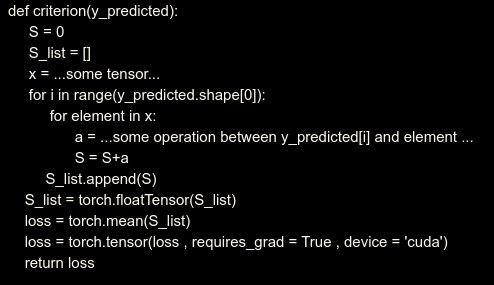# Gradient of output is 0

I am sorry I cannot share my code, but is it somewhere along the lines of:When I try to print the gradient of y_predicted (which is the output label) in my training loop after calling .backward(), it gives me None. Can anyone help with where I am going wrong ?

P.S. Please ignore the logic errors in the code.

Don’t use torch.tensor in between and never use torch.FloatTensor, but instead use `stack` to make a list of tensors into one large tensor.
Of course, the presentation makes me wonder if you can cut the for loops by vectorizing and cumsum, too.

Best regards

Thomas

Here is an axample code.
1)loss function

2)training loop

This is an example code for something that I want to do. Here when i print output_label.grad , it returns None. I don’t get what I am doing wring here. Also, can you please give an example about how can i get rid of the for loops ?

It says that output_label is not a leaf node of the graph. What does this mean ? If I am not wrong, while doing backpropagation, the gradient of the loss with respect to the output also needs to be calculated. uu here, output_label.grad is giving None.
Am I doing something wrong here ?

Under the hood, yes, the autograd engine does compute the gradient of the loss with respect to the output but the result is never stored but passed along backward through the graph to compute the gradient with respect to the leaves.
What are leaves: that’s exactly the tensors created with `requires_grad=True`.
Typically, that would be the parameters of your `net` and you don’t really need to explicitly set `requires_grad=True` because that’s the default for parameters.

That’s why you never really want to write `z_predicted = torch.tensor(z_predicted,requires_grad=True)` as it breaks your autograd graph.

If you really just gradient of your loss with respect to `output_label`, try:

``````output_label = net(input)
output_label_leaf = output_label.detach()